# Objective 4. Construct a Formula and Use the Sum Function

Excel performs calculations on numbers; that's why you use Excel. If you make changes to the numbers, Excel automatically re-calculates. This is one of the most powerful and valuable features of Excel. You can arrange data in a format of columns and rows in other application programsin a word processing program, for exampleand even perform simple calculations. Excel, however, performs complex calculations on numbers.

Recall that a cell contains either a value or a formula. A formula is an equation that directs Excel to perform mathematical calculations on values in other cells, and to place the result in the cell containing the formula. You can construct your own formulas, or you can use one of Excel's prebuilt formulas called a function.

Activity 1.9. Constructing a Formula and Using the Sum Function

In this activity, you will sum the monthly purchases for tableware at the restaurant in Dallas.

 1. Click cell B8 to make it the active cell and press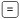. Compare your screen with Figure 1.41.   Figure 1.41.The equal sign (=) displays in the cell with the insertion point blinking, ready to accept more data. All formulas begin with the = sign, which is the signal that directs Excel to begin a calculation. The Formula Bar displays the = sign, and the Formula Bar Cancel and Enter buttons display. 2. At the insertion point, type b3 and then compare your screen with Figure 1.42.   Figure 1.42.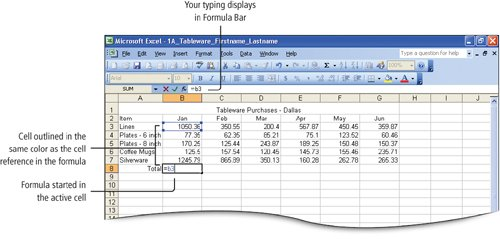You can use uppercase or lowercase letters in a cell reference. Cell B3 is surrounded by a blue border with small corner boxes. This indicates that the cell is part of an active formula. The color used in the box matches the color of the cell reference in the formula. 3. At the insertion point, press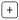(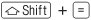) and then type b4 Alternatively, use thekey on your numeric keypad, which does not require the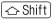key. A border of another color surrounds cell B4, and the color matches the color of the cell reference in the active formula. Recall that when typing cell references, it is not necessary to use uppercase letters. 4. At the insertion point, type +b5+b6+b7 and then press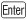. The result of the formula calculation2669.25displays in the cell. If your total is not the same, review the numbers you typed in cells B3:B7 and correct any errors you may have made when entering the data. 5. Click cell B8 again to make it the active cell and look at the Formula Bar. Compare your screen with Figure 1.43.   Figure 1.43. (This item is displayed on page 622 in the print version)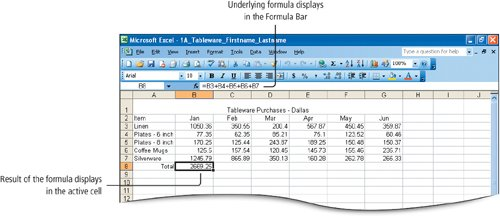You created a formula that adds the values in cells B3 through B7, and the result of adding the values in those cells displays in cell B8. Although cell B8 displays the result of the formula, the formula itself is displayed in the Formula Bar. This is referred to as the underlying formula. Always view the Formula Bar to be sure of the exact content of a cella displayed number might actually be a formula. 6. In cell C8, type = to signal the beginning of a formula. Then, point to cell C3, click once, and compare your screen with Figure 1.44.   Figure 1.44.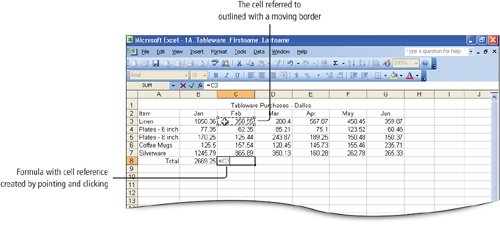The reference to the cell, C3, is added to the active formula. A moving border surrounds the cell referred to, and the border color and the color of the cell reference in the formula are color coded to match. 7. At the insertion point, press thekey and then click C4. Repeat this process to complete the formula to add cells C3 through C7 and then press. The result of the formula calculation1561.77displays in the cell. This method of constructing a formula is the point and click method. For left-handed individuals, this is efficient because you can click cells with the mouse using your left hand while pressing the math operator keys and thekey on the numeric keypad with your right hand. 8. Select cell D8. On the Standard toolbar, click the AutoSum button, and then compare your screen with Figure 1.45.   Figure 1.45.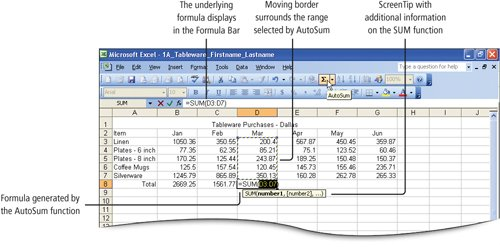AutoSum is an Excel functiona predefined formula. Cells D3:D7 are surrounded by a moving border, and =SUM(D3:D7) displays in cell D8. The = sign signals the beginning of a formula, SUM indicates the type of calculation that will take place (addition), and (D3:D7) indicates the range of cells on which the sum operation will be performed. A ScreenTip provides additional information about the action. 9. Look at the Formula Bar, and notice that the formula also displays there. Then, look again at the cells surrounded by the moving border. When the AutoSum function is activated, Excel first looks above the active cell for a range of cells to sum. If no range is above the active cell, Excel will look to the left for a range of cells to sum. Regardless, Excel will propose a range of cells to sum, and if the proposed range is not what you had in mind, you can select a different group of cells. 10. Press. The sum of Tableware Purchases for March1000.06displays in cell D8. Because the AutoSum function is so frequently used, it has its own button on the Standard toolbar. As you progress in your study of Excel, you will use additional Excel functions. 11. Select the range E3:G8 and then compare your screen with Figure 1.46.   Figure 1.46. (This item is displayed on page 624 in the print version)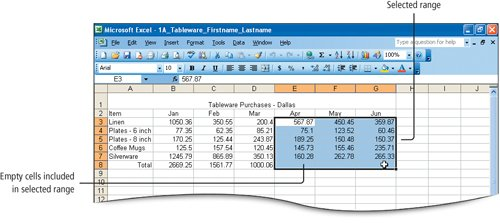By selecting a range of cells and including the empty cells at the bottom of each column, you can apply the AutoSum function to sum several columns at once; the formula for adding each column will be placed in the empty cells at the bottom of each column. 12. On the Standard toolbar, click the AutoSum button. An AutoSum formula and displayed result displays in cells E8, F8, and G8 indicating the sums 1138.23, 1142.69, and 1071.74. 13. Click cell E8 and notice the formula in the Formula Bar. Click cell F8 and G8 to see the formula in the Formula Bar. The cells display the result of the calculated function, which is a formula that Excel has prebuilt and named. 14. On the Standard toolbar, click the Save button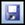to save the changes you have made to your workbook.

[Page 624 (continued)]

### Objective 5 Format Data and Cells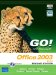GO! with Microsoft Office 2003 Brief (2nd Edition)
ISBN: 0131878646
EAN: 2147483647
Year: 2004
Pages: 448